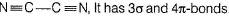Courses

# Test: Interhalogens And Pseudo Halogens

## 25 Questions MCQ Test Chemistry Class 12 | Test: Interhalogens And Pseudo Halogens

Description
This mock test of Test: Interhalogens And Pseudo Halogens for Class 12 helps you for every Class 12 entrance exam. This contains 25 Multiple Choice Questions for Class 12 Test: Interhalogens And Pseudo Halogens (mcq) to study with solutions a complete question bank. The solved questions answers in this Test: Interhalogens And Pseudo Halogens quiz give you a good mix of easy questions and tough questions. Class 12 students definitely take this Test: Interhalogens And Pseudo Halogens exercise for a better result in the exam. You can find other Test: Interhalogens And Pseudo Halogens extra questions, long questions & short questions for Class 12 on EduRev as well by searching above.
QUESTION: 1

### Direction (Q. Nos. 1-10) This section contains 10 multiple choice questions. Each question has four choices (a), (b), (c) and (d), out of which ONLY ONE is correct. Q.  Which of the following is not a pseudo halideion?

Solution:

Pseudo halides are uni-negative charged similar to halideions.

QUESTION: 2

### The stability of interhalogen compounds follows the order

Solution:

Electropositive character of halogens is in order I > Br > Cl . Central atom is bigger in size, more electropositive nature form stable interhalogen compound.

QUESTION: 3

### Match the following molecules or ions to their shapes. Which pairing is correct?

Solution:

[I3]= Linear ; BrF= Square pyramidal; ClF3 = 'T' shaped

QUESTION: 4

In XA5, the central atom has (both X and A are halogens)

Solution:

It has square pyramidal shape and it has 5 bond pairs and one lone pair.

QUESTION: 5

Which of the following pairs is isostructural?

Solution:

Both have sp3d2-hybridisation with sq uare pyramidal shape

QUESTION: 6

Which of the following pairs shows correct match between formula and structure of a species?

Solution:

[BrF2]= sp3, angular:
[ICI4]- = sp3d2, square planar;
IF5 = sp3d2, sqare pyramidal;
BrF3 = sp3d, T shaped

QUESTION: 7

HClO is known as

Solution:
QUESTION: 8

Which of the following is correct for CsBr3?

Solution:

CsBr3 contains Cs+, Br- and lattice Br2 molecules respectively.

QUESTION: 9

In the known interhalogen compounds, the maximum number of atoms are

Solution:

In IF7, iodine is the least electronegative halogen, so its higher oxidation number (+7) is more stable than those of the lighter member of the group

QUESTION: 10

Identify the interhalogen compound with zero dipole moment,

Solution:

Due to pentagonal bipyramidal geometry, IF7 has zero dipole momen

*Multiple options can be correct
QUESTION: 11

Direction (Q. Nos. 16-20) This section contains 5 multiple choice questions. Each question has four choices (a), (b), (c) and (d), out of which ONE or MORE THAN ONE are correct.

Q.

Identify the products formed at the electrodes upon electrolysis of molten ICI.

Solution: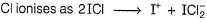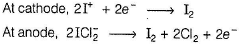*Multiple options can be correct
QUESTION: 12

When Cs[ICl2] undergo thermal dissociation, the products formed are

Solution:

When a polyhalide containing more than one type of halogen atom undergo thermal dissociation, the metal monohalide is always formed, which has highest lattice energy. In this, CsCI has more lattice energy than Csl.

*Multiple options can be correct
QUESTION: 13

Pseudo halogens among the following are

Solution:

(CN)2, (OCN)2 and (SeCN)2 are pseudo halogens.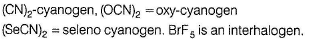*Multiple options can be correct
QUESTION: 14

Which has both ionic and covalent bonding?

Solution: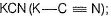KI3(I—K . I—I); CsBr3(Br—Cs . Br—Br) all three have both ionic as well as covalent bonds But K2O (K—O—K) has only ionic bonds.

*Multiple options can be correct
QUESTION: 15

Same hybridisation is present for the central atom in

Solution: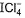and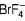both have sp3d2-hybridisation.

QUESTION: 16

Direction (Q. Nos. 16 and 17) This section contains a paragraph, each describing theory, experiments, data, etc. Two questions related to the paragraph have been given. Each question has only one correct answer among the four given options (a), (b), (c) and (d).

Passage

When two different halogens react with each other, the compounds formed are called interhalogen compounds.
The general formula of these compounds is given by XX',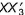,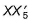and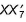.
Here, X = halogen of large size and more electropositive.
X' = halogen of smaller size and more electronegative,
e.g. CIF, ClF3, IF5, IF7, etc.
All are covalent and diamagnetic.
They are either volatile liquids or solids except CIF, BrF, CIF3, IF5 and IF7.
Interhalogen compounds are more reactive than halogens (except fluorine). The reason is X—X' bond in interhalogens is weaker than X—X bond in halogens (except F— F bond).
Hydrolysis of interhalogens Interhalogen compounds undergo hydrolysis giving halide ion derived from the smaller halogen and a hypohalite (when XX'), halite (when), halate (when) and perhalate (when) n ion derived from the larger halogen.

XX' + H2O → HX + HOX

Q.

Which is more stable?

Solution:

Thermal stability of diatomic interhalogen increases with increase in difference between the electronegativities of the halogen atoms.
Hence, increasing order of the stability of given interhalogens (in options) will be
BrCI < CIF < BrF < IF

QUESTION: 17

When two different halogens react with each other, the compounds formed are called interhalogen compounds.
The general formula of these compounds is given by XX',,and.
Here, X = halogen of large size and more electropositive.
X' = halogen of smaller size and more electronegative,
e.g. CIF, ClF3, IF5, IF7, etc.
All are covalent and diamagnetic.
They are either volatile liquids or solids except CIF, BrF, CIF3, IF5 and IF7.
Interhalogen compounds are more reactive than halogens (except fluorine). The reason is X—X' bond in interhalogens is weaker than X—X bond in halogens (except F— F bond).
Hydrolysis of interhalogens Interhalogen compounds undergo hydrolysis giving halide ion derived from the smaller halogen and a hypohalite (when XX'), halite (when), halate (when) and perhalate (when) n ion derived from the larger halogen.

XX' + H2O → HX + HOX

Q.

BrCI on hydrolysis produce

Solution:

BrCL+ H2O → HOBr + HCL

QUESTION: 18

Direction (Q. Nos. 18 and 19) This section is based on Statement I and Statement II. Select the correct answer from the codes given below.

Q.

Statement I : Interhalogens are ionic compounds.

Statement II : Greater the difference in electronegativity of two different halogen atoms, more is the stability of their interhalogen compound.

Solution:

Interhalogens are covalent compounds.

QUESTION: 19

Statement I : Cyanide ions (CN-) are also called pseudo halogens.

Statement II : Cyanide ions make electrovalent bonds with electropositive elements like Na, K, etc.

Solution:

Pseudo halogens have similar chemical properties as that of halogens like complex formation.

QUESTION: 20

Direction (Q. No. 20) Choices fo r the correct combination of elements from Column I and Column II are given as options (a), (b), (c) and (d), out of which one is correct.

Q.

Match the Column I with Column II and mark the correct option from the codes given below.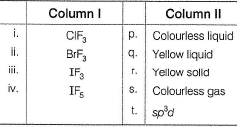Solution: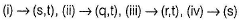*Answer can only contain numeric values
QUESTION: 21

Direction (Q. Nos. 21-25) This section contains 5 questions. When worked out will result in an integer from 0 to 9 (both inclusive).

Q.

How many pseudo halide ions are there, among the given ions?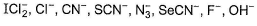Solution: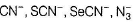*Answer can only contain numeric values
QUESTION: 22

In CIF3, if both lone pairs occupy axial positions then find the number of lone pair-bond pair repulsions.

Solution:

In this geometry, each lone pair is 90° with the bond pairs. Hence, lone pair-bond pair repulsions are six.

*Answer can only contain numeric values
QUESTION: 23

How many of the given structures are isostructural with XeF4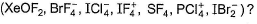Solution: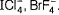*Answer can only contain numeric values
QUESTION: 24

Find the sum of bond pairs and non-bonding electron pairs in ICI molecule.

Solution:

3 lone pairs and 1 bond pair.

*Answer can only contain numeric values
QUESTION: 25

Find the total number of covalent bonds present in cyanogens.

Solution: